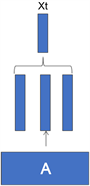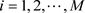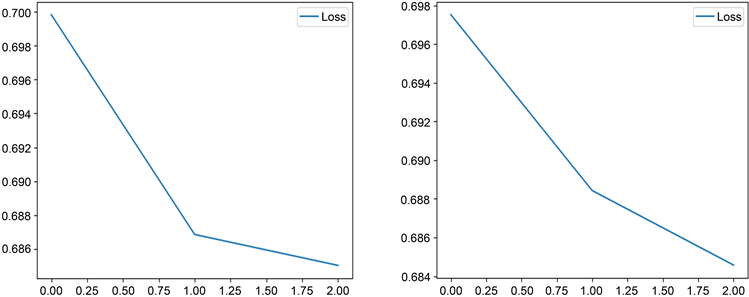# 基于电子病历的疾病风险预测Disease Risk Prediction Based on EHR

DOI: 10.12677/HJDM.2020.101005, PDF, HTML, XML, 下载: 184  浏览: 1,160  国家自然科学基金支持

Abstract: Data driven health care, as the use of available large-scale medical data, to provide the best and most personalized care, is becoming one of the main trends of the success of the revolution in the medical industry. Electronic health record is the main carrier to promote the success of this da-ta-driven medical revolution. In this paper, we use the method of deep learning, based on the word embedding model to express the EHR information, and use the characteristics of the long-term memory network model to solve the irregular time of EHR information and the long-term depend-ence of disease information, so as to achieve the prediction of disease risk. Compared with the con-volution neural network model, the results show the effectiveness of this method.

1. 引言

2. 相关工作

3. 基本概念

3.1. 词表示

3.2. 学习词嵌入矩阵

$h=\frac{{E}^{{w}_{i-C}}+\cdots +{E}^{{w}_{i-1}}+{E}^{{w}_{i+1}}+\cdots +{E}^{{w}_{i+C}}}{2C}$ (1)

$P\left({w}_{i}|{w}_{i-C},\cdots ,{w}_{i-1},{w}_{i+1},\cdots ,{w}_{i+C}\right)=\text{softmax}\left(a\right)$ (2)

$L=\frac{1}{T}\underset{i=1}{\overset{T}{\sum }}\mathrm{log}P\left({w}_{i}|{w}_{i-C},\cdots ,{w}_{i-1},{w}_{i+1},\cdots ,{w}_{i+C}\right)$ (3)

3.3. 长短期记忆网络(LSTM)

${i}_{t}=\sigma \left({W}_{i}{x}_{t}+{U}_{i}{h}_{t-1}+{b}_{i}\right)$ (4)

${f}_{t}=\sigma \left({W}_{f}{x}_{t}+{U}_{f}{h}_{t-1}+{b}_{f}\right)$ (5)

${o}_{t}=\sigma \left({W}_{o}{x}_{t}+{U}_{o}{h}_{t-1}+{b}_{0}\right)$ (6)

${g}_{t}=\mathrm{tanh}\left({W}_{c}{x}_{t}+{U}_{c}{h}_{t-1}+{b}_{c}\right)$ (7)

${c}_{t}={f}_{t}\ast {c}_{t-1}+{i}_{t}\ast {g}_{t}$ (8)

${h}_{t}={o}_{t}\ast \mathrm{tanh}\left({c}_{t}\right)$ (9)

3.4. 模型复杂性

LSTM层的参数：

4. 基于电子病历的风险预测模型

4.1. 可变大小的入院信息的表示Figure 1. Code embedding

4.2. 池化

${x}_{t}^{i}$ 是向量 ${x}_{t}$ 的第i个元素，入院采用最大池化、合并池化或平均池化，合并方式如下：

${x}_{t}^{i}=\mathrm{max}\left({A}_{i}^{{d}_{1}},{A}_{i}^{{d}_{2}},\cdots ,{A}_{i}^{{d}_{h}}\right)$

$i=1,2,\cdots ,M$。这类似于选择性注意诊断和干预之间的影响最大的因素。它也类似于通常的编码实践，即选择一个诊断作为入院的主要原因。

${x}_{t}^{i}=\frac{{A}_{i}^{{d}_{1}}+{A}_{i}^{{d}_{2}}+\cdots +{A}_{i}^{{d}_{h}}}{\sqrt{|{A}_{i}^{{d}_{1}}+{A}_{i}^{{d}_{2}}+\cdots +{A}_{i}^{{d}_{h}}|}}$。归一化降低了大量的诊断和干预的影响。

${x}_{t}=\frac{{A}^{{d}_{1}}+{A}^{{d}_{2}}+\cdots +{A}^{{d}_{h}}}{h}$

4.3. LSTM预测模型

${\stackrel{^}{y}}_{p}=\text{softmax}\left({W}_{y}{z}^{\left(p\right)}+{b}_{y}\right)$ (10)

$\theta$ 为LSTM模型中所有参数的集合，预测概率向量 ${\stackrel{^}{y}}_{p}$ 也可用模型后验分布 $P\left({y}_{p}|{X}^{p};\theta \right)$ 表示，其中 ${y}_{p}$ 是真值。利用真值 ${y}_{p}$ 与预测概率 ${\stackrel{^}{y}}_{p}$ 之间的交叉熵来计算损失，因此，风险预测的目标函数是交叉熵的平均值：

$L\left(\theta \right)=-\frac{1}{|P|}\underset{p=1}{\overset{|P|}{\sum }}\left({y}_{p}^{T}\mathrm{log}\left({\stackrel{^}{y}}_{p}\right)+{\left(1-{y}_{p}\right)}^{T}\mathrm{log}\left(1-{\stackrel{^}{y}}_{p}\right)\right)$ (11)

5. 实验

5.1. 实验设置Table 1. Parameter settings on the prediction model LSTMTable 2. Parameter settings on prediction model CNN

5.2. 实验结果与分析Figure 2. Loss image of CCN and LSTM modelsTable 4. Performance evaluation of the three models

6. 结论与展望

  Jensen, P.B., Jensen, L.J. and Brunak, S. (2012) Mining Electronic Health Records: Towards Better Research Applica-tions and Clinical Care. Nature Reviews Genetics, 13, 395-405. https://doi.org/10.1038/nrg3208  Caroprese, L., Veltri, P., Vocaturo, E. and Zumpano, E. (2018) Deep Learning Techniques for Electronic Health Record Analysis. 2018 9th International Conference on Information, Intelligence, Systems and Applications, Zakynthos, Greece, 23-25 July 2018, 1-4. https://doi.org/10.1109/IISA.2018.8633647  Madsen, L.B. (2014) Data-Driven Healthcare: How Analytics and BI Are Transforming the Industry. MIT Technology Review Business Report, 117, 1-19.  Madsen, L.B. (2014) Data-Driven Healthcare: How Analytics and BI Are Transforming the Industry. Wiley, New York.  Ho, J.C., Ghosh, J. and Sun, J.M. (2014) Marble: High-Throughput Phenotyping from Electronic Health Records via Sparse Nonnegative Tensor Factorization. In: Proceedings of the 20th ACM SIGKDD International Conference on Knowledge Discovery and Data Dining (KDD’14), 115-124.  Cheng, Y., Wang, F., Zhang, P. and Hu, J.Y. (2016) Risk Pre-diction with Electronic Health Records: A Deep Learning Approach. Proceedings of the 2016 SIAM International Con-ference on Data Mining (SDM’ 16), Miami, FL, 5-7 May 2016, 432-440. https://doi.org/10.1137/1.9781611974348.49  Zhou, J., Wang, F., Hu, J. and Ye, J. (2014) From Micro to Macro: Data Driven Phenotyping by Densification Longitudinal Electronic Medical Records. In: Proceedings of the 20th ACM SIGKDD International Conference on Knowledge Discovery and Data Mining, ACM, New York, 135-144. https://doi.org/10.1145/2623330.2623711  Wang, F., Zhang, P., Qian, B., Wang, X. and Davidson, I. (2014) Clinical Risk Prediction with Multilinear Sparse Logistic Regression. In: Proceedings of the 20th ACM SIGKDD Inter-national Conference on Knowledge Discovery and Data mining, ACM, New York, 145-154. https://doi.org/10.1145/2623330.2623755  Chen, L. (2019) Assertion Detection in Clinical Natural Language Pro-cessing: A Knowledge-Poor Machine Learning Approach. 2019 IEEE 2nd International Conference on Information and Computer Technologies, Kahului, HI, 14-17 March 2019, 37-40. https://doi.org/10.1109/INFOCT.2019.8710921  Hochreiter, S. and Schmidhuber, J. (1997) Long Short-Term Memory. Neural Computation, 9, 1735-1780. https://doi.org/10.1162/neco.1997.9.8.1735  Rumelhart, D.E. (1986) Learning Representations by Back-Propagating Errors. Nature, 323, 533-536. https://doi.org/10.1038/323533a0  Choi, E., Bahadori, M.T., Searles, E., et al. (2016) Multi-Layer Representa-tion Learning for Medical Concepts. Proceedings of the 22nd ACM SIGKDD International Conference on Knowledge Discovery and Data Mining, August 2016, 1495-1504. https://doi.org/10.1145/2939672.2939823  Choi, E., Bahadori, M., Song, L., et al. (2016) GRAM: Graph-Based Attention Model for Healthcare Representation Learning. Computer Science, 787-795.  Ma, F.L., Radha, C. and Zhou, J. (2017) Dipole: Diagnosis Prediction in Healthcare via Attention-based Bidirectional Recurrent Neural Networks. Proceedings of the 23rd ACM SIGKDD International Conference on Knowledge Discovery and Data Mining, August 2017, 1903-1911. https://doi.org/10.1145/3097983.3098088  Che, Z., Cheng, Y., Zhai, S., Sun, Z. and Liu, Y. (2017) Boosting Deep Learning Risk Prediction with Generative Adversarial Networks for Electronic Health Records. 2017 IEEE Inter-national Conference on Data Mining, New Orleans, LA, 18-21 November 2017, 787-792. https://doi.org/10.1109/ICDM.2017.93  Choi, E., Bahadori, M.T., Kulas, J.A., Schuetz, A. and Stewart, W.F. (2016) RETAIN: An Interpretable Predictive Model for Healthcare Using Reverse Time Attention Mechanism. Computer Science, 3504-3512.  Suo, Q., Ma, F., Yuan, Y., et al. (2017) Personalized Disease Prediction Using a CNN-Based Similarity Learning Method. 2017 IEEE International Conference on Bioinformatics and Biomedicine, Kansas City, MO, 13-16 November 2017, 811-816. https://doi.org/10.1109/BIBM.2017.8217759